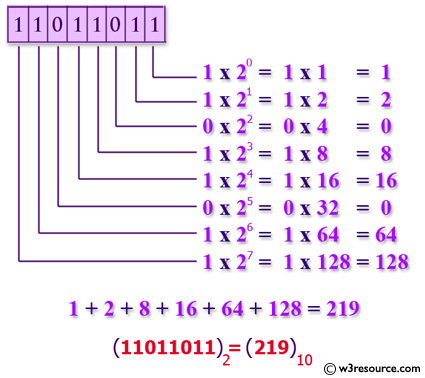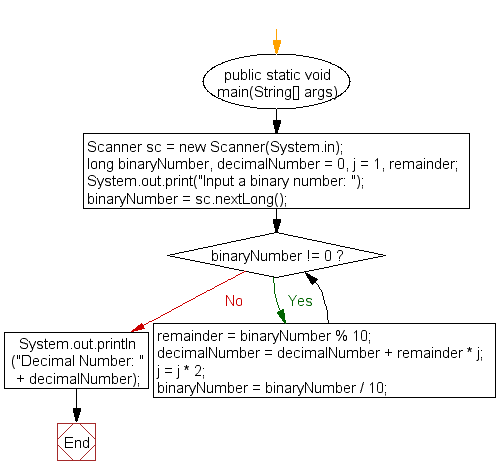﻿ Java exercises: Convert a binary number to decimal number - w3resource# Java Exercises: Convert a binary number to decimal number

## Java Basic: Exercise-22 with Solution

Write a Java program to convert a binary number to decimal number.

Binary number: A binary number is a number expressed in the base-2 numeral system or binary numeral system. This system uses only two symbols: typically 1 (one) and 0 (zero).

Decimal number: The decimal numeral system is the standard system for denoting integer and non-integer numbers. It is also called base-ten positional numeral system.

Pictorial Presentation: Binary to Decimal numberSample Solution:

Java Code:

``````import java.util.Scanner;
public class Exercise22 {
public static void main(String[] args)
{
Scanner sc = new Scanner(System.in);
long binaryNumber, decimalNumber = 0, j = 1, remainder;
System.out.print("Input a binary number: ");
binaryNumber = sc.nextLong();

while (binaryNumber != 0)
{
remainder = binaryNumber % 10;
decimalNumber = decimalNumber + remainder * j;
j = j * 2;
binaryNumber = binaryNumber / 10;
}
System.out.println("Decimal Number: " + decimalNumber);
}
}
```
```

Sample Output:

```Input a binary number: 100
Decimal Number: 4
```

Flowchart:Java Code Editor:

What is the difficulty level of this exercise?

Test your Programming skills with w3resource's quiz.

﻿

## Java: Tips of the Day

countOccurrences

Counts the occurrences of a value in an array.

Use Arrays.stream().filter().count() to count total number of values that equals the specified value.

```public static long countOccurrences(int[] numbers, int value) {
return Arrays.stream(numbers)
.filter(number -> number == value)
.count();
}
```

Ref: https://bit.ly/3kCAgLb